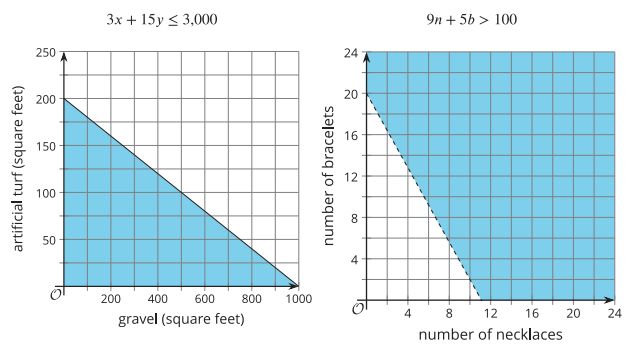# Week 15 Day 1 Lesson SummaryHow are the solution regions of the two inequalities alike?

How do we know where the boundary line would be for each graph?

For each inequality, how did we find out which side of the line contains the solutions?

In the first situation, some pairs of values that are in the solution region don't make sense in the situation. Can you explain why a pair such as (800,1), which is in the solution region, might not be a reasonable option for the homeowner?

In the second situation, we know that fractional values are not meaningful even though they are in the shaded region. Can you think of other reasons that some points in the solution region might not make sense?